# Wich one of the following carbonyl compounds cannot be prepared by

Question:

Wich one of the following carbonyl compounds cannot be prepared by addition of wate on an alkyne in the presence of $\mathrm{HgSO}_{4}$ and $\mathrm{H}_{2} \mathrm{SO}_{4}$ ?

1.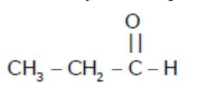2.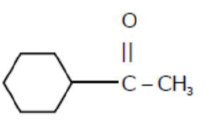3.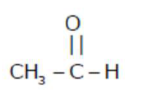4.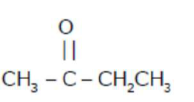Correct Option: 1

Solution:

Reaction of Alkyne with $\mathrm{HgSO}_{4} \& \mathrm{H}_{2} \mathrm{SO}_{4}$ follow as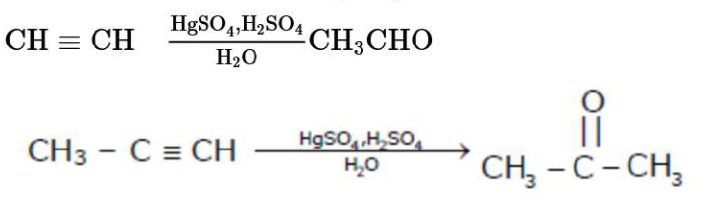Hence, by this process preparation of $\mathrm{CH}_{3} \mathrm{CH}_{2} \mathrm{CHO}$Cann't possible.